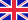•Fibonacci-Like Sequence

Autor: Mohsen Dar

In the recent years,there has been much interest in the field of Fibonacci numbers.The Fibonacci sequence is considered as one of the most interesting sequences in all of mathematics.There are several ways in which the Fibonacci sequence can be generalized.... Viac o knihe

Na objednávku, dodanie 2-4 týždne

42.84 €

bežná cena: 50.40 €

O knihe

In the recent years,there has been much interest in the field of Fibonacci numbers.The Fibonacci sequence is considered as one of the most interesting sequences in all of mathematics.There are several ways in which the Fibonacci sequence can be generalized. In this book,the Fibonacci-Like sequence has been generalized.The associated initial conditions are the sum of initial conditions of Fibonacci sequence and m times the initial conditions of Lucas sequence respectively.Various properties and identities have been developed for this Generalized Fibonacci-Like sequence.

• Vydavateľstvo: LAP Lambert Academic Publishing
• Rok vydania: 2015
• Formát: Paperback
• Rozmer: 220 x 150 mm
• Jazyk: Anglický jazyk
• ISBN: 9783659197512

Generuje redakčný systém BUXUS CMS spoločnosti ui42.